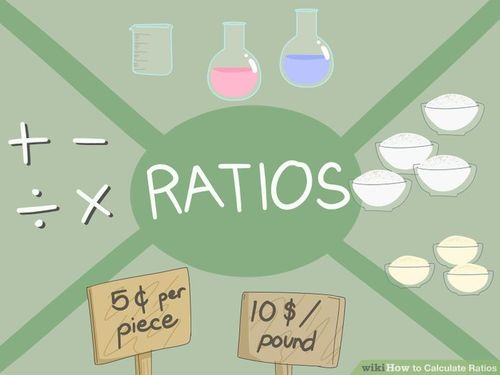Ratios
3 years ago
ahintze
Save
Edit
Host a game
Live GameLive
Homework
Solo Practice
Practice10 QuestionsShow answers
• Question 1
30 seconds
Q. A ratio is...
the difference between two numbers.
a comparison of two numbers by division.
a comparison of two numbers by multiplication.
the sum of two numbers.
• Question 2
30 seconds
Q. Tell if the ratio is part-to-part or part-to-whole.
4 apples to 3 oranges
Part-to-Part
Part-to-Whole
• Question 3
30 seconds
Q. Tell if the ratio is part-to-part or part-to-whole.
10 questions : 3 incorrect
Part-to-Part
Part-to-Whole
• Question 4
30 seconds
Q. Tell if the ratio is part-to-part or part-to-whole.
In a a mini bag of M&Ms, there are 16 M&Ms and 5 of them are red.
Part-to-Part
Part-to-Whole
• Question 5
30 seconds
Q. Tell if the ratio is part-to-part or part-to-whole.
4 inches of snow on Tuesday to 6 inches of snow on Friday
Part-to-Part
Part-to-Whole
• Question 6
30 seconds
Q. Write the ratio as a fraction:  20 people RSVP'd to the party out of 30 invitees.
½
• Question 7
30 seconds
Q. Write the ratio as a fraction:  4:16
¾
¼
• Question 8
30 seconds
Q. Write the ratio as a colon:
8:5
10:18
5:8
12:4
• Question 9
30 seconds
Q. Which ratio is greater?
4:1 or 6:2